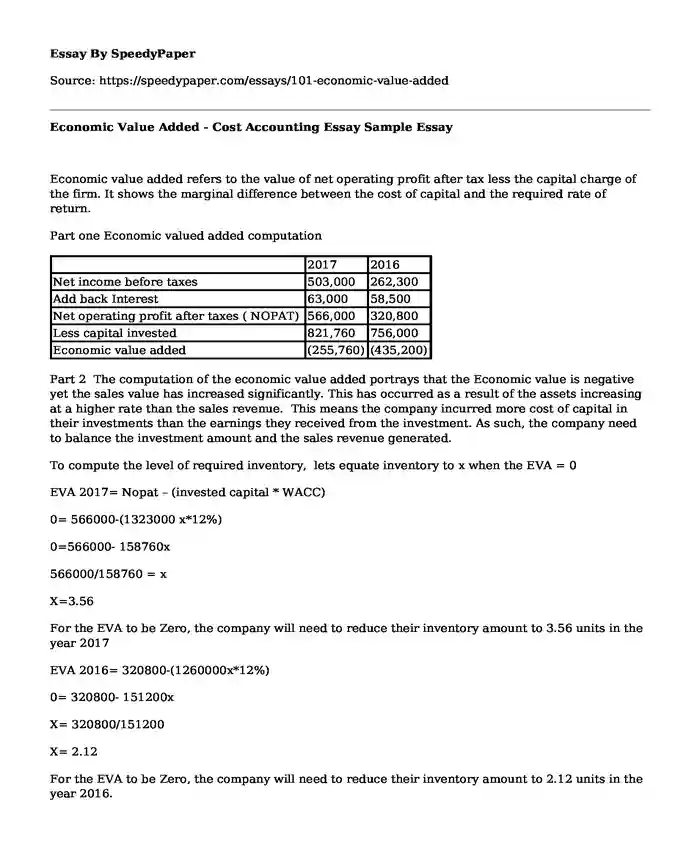# Economic Value Added - Cost Accounting Essay Sample

Published: 2018-04-20Type of paper: Essay Categories: Economics Finance Sales Accounting Pages: 2 Wordcount: 353 words
143 views

Economic value added refers to the value of net operating profit after tax less the capital charge of the firm. It shows the marginal difference between the cost of capital and the required rate of return.

Is your time best spent reading someone else’s essay? Get a 100% original essay FROM A CERTIFIED WRITER!

Part one Economic valued added computation

 2017 2016 Net income before taxes 503,000 262,300 Add back Interest 63,000 58,500 Net operating profit after taxes ( NOPAT) 566,000 320,800 Less capital invested 821,760 756,000 Economic value added (255,760) (435,200)

Part 2 The computation of the economic value added portrays that the Economic value is negative yet the sales value has increased significantly. This has occurred as a result of the assets increasing at a higher rate than the sales revenue. This means the company incurred more cost of capital in their investments than the earnings they received from the investment. As such, the company need to balance the investment amount and the sales revenue generated.

To compute the level of required inventory, lets equate inventory to x when the EVA = 0

EVA 2017= Nopat – (invested capital * WACC)

0= 566000-(1323000 x*12%)

0=566000- 158760x

566000/158760 = x

X=3.56

For the EVA to be Zero, the company will need to reduce their inventory amount to 3.56 units in the year 2017

EVA 2016= 320800-(1260000x*12%)

0= 320800- 151200x

X= 320800/151200

X= 2.12

For the EVA to be Zero, the company will need to reduce their inventory amount to 2.12 units in the year 2016.

Part 3

EVA 2017= Nopat – (invested capital * WACC) (Horngren, 2009).

0= 566000-(1323000-750000) x*12%)

0=566000-1248000x*12%

0=566000- 149760x

566000/149760= x

X=3.78 units

Thus the company need to hold 3.78 units of inventory for the EVA to be zero in 2017.

EVA 2016= 320800-(1260000-75000) x*12%)

0= 320800- 142200x

X= 320800/142200

X= 2.26

Thus the company need to hold 2.26 units of inventory for the EVA to be zero in 2016

References

Horngren, C. T. (2009). Cost accounting: A managerial emphasis, 13/e. Pearson Education India.